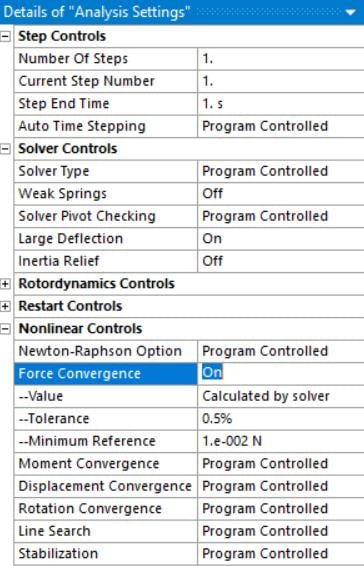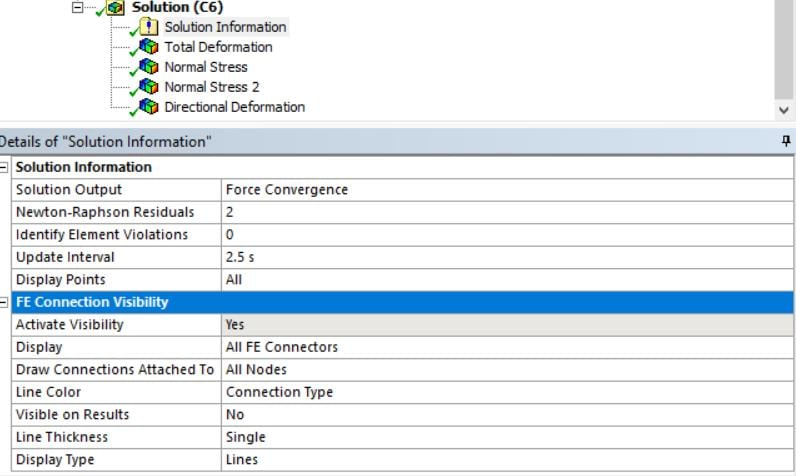## General Mechanical

•skpb
Subscriber

The first three images depicts the model and its boundary conditions(B.C.)

• model with fixed face B.C.

• model with Force applied on the other face

• model with Preloaded bolt B.C

The next two images shows Convergence plots

• Force Convergence

• Displacement Convergence

The analysis settings are

• External force applied= 100 Newton

Note:  The solution is converged

Question 1: "How to read the force convergence plot?"

I do understand that its non-linear behavior so there is no exact solution, we should approximate with the values which gives residuals less than convergence, for this Newton Raphson method is used and it is done in iterations. But what i don't get are

• What is Force criterion?

Is it the external applied force?, if it is but i have applied 100 N as external force on the model, but it is showing near 1 N also each load steps it should increase and reach towards 100 right?

• What is Force convergence?

Is it the internal forces in each step.

Question 2: "How to read the displacement convergence plot?"

Same doubts as Force convergence plot

Thanks

•peteroznewman
Subscriber

From the ANSYS Help system.

Convergence Criterion

When solving nonlinear steady-state, static, or transient analyses, an iterative procedure (equilibrium iterations) is carried out at each substep. Successful solution is indicated when the out-of-balance loads are less than the specified convergence criteria. Criteria appropriate for the analysis type and physics are displayed in this grouping. Convergence controls are “step aware”. This means that the setting can be different for each step.

ANSYS computes a Force convergence criteria for you. When you leave it Program Controlled, the value is 0.5% but you can override that if you have good reason to do so.Displacement convergence is also 0.5% if left Program Controlled, and you can override this also.

•skpb
Subscriber

hello, I have small difficulties to see which elements are creating problems in convergence. Could u explain how to get it? Though I have changed my Newton Raphson Residual under solution information to 2.•peteroznewman
Subscriber

Your problem converged. No elements were a problem.

•skpb
Subscriber

Yeah !!! Thanks for the Help